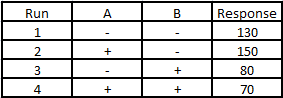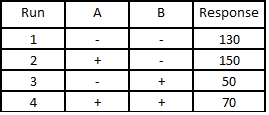India - +91 7838547711, US - +1 513 657 9333

## Lean Six Sigma Black Belt Sample Paper 2: Improve

1. The operators of a manufacturing cell work out a more orderly arrangement for tool storage and establish a schedule to maintain cleanliness daily. These improvements are best described by which approach to problem solving?
2. Power of the design can be increased by:
3. The correct sequence in a DoE study is
4. In a fractional factorial design, the 2 factor interactions (2FI) are confounded with other 2FI’s. What is the resolution of this design
5. Two drugs A & B were evaluated for their effectiveness. However, drug A was tested only in individuals with <30 years age and drug B was tested only in individuals with >30 years age. In such a case the effect of the drugs and age of the individuals is said to be
6. Calculate the effect of factor “B” if7. One of the following is not correctly matched to its purpose
8. If the effect of factor A is 10, it means
9. With respect to DoE, “Block” for_________ factors and “Randomize” for__________factors
10. The disadvantage of using Pugh Matrix is:
11. Hindering CTQ’s from HoQ can be optimized to achieve the “Sweet spot” using: (Sweet Spot: Targets for multiple CTQ’s are achieved):
12. To check if the relationship between X & Y exhibits curvature (non-linearity) we introduce_______in the design
13. Determine the number of runs that would be required if we had 3 factors, 2 levels, 2 replicates of the corner points, 3 Center points and 1 block.
14. All the pairs below are incorrectly matched, EXCEPT
15. One of the following DoE can be preferably used as a screening design?
16. Which of the following is an example of a pilot?
17. With respect to an interaction existing between two factors, one of the following is TRUE about the interaction plot:
18. To assess the significance of factors in either a fractional or a full-factorial design, a black belt can use the_________in Minitab
19. One factor at a time studies would not be able to identify interactions between the factors as compared to full factorial designs. The statement is:
20. Statements given below are true, EXCEPT:
21. Calculate the interaction effect (AB) for the following data and match with the appropriate statement22. What resolution will you choose to perform a two-level fractional factorial design; 6 factors, 2 replicates necessary; if the budget doesn’t allow more than 20 experiments
23. All the following statements regarding reduction of a model for 3 factors (A,B & C) are true, EXCEPT:
24. One of the following statements is incorrect:
25. Residual is an estimate of experimental error obtained by subtracting the ________ from the_________.
26. An experimental run is conducted several times for the same treatment combination to neutralize the effect of any uncontrollable, unknown factor. This is called___________. However, conducting repeated runs at the same experimental conditions (with a time gap in most cases) is called__________.